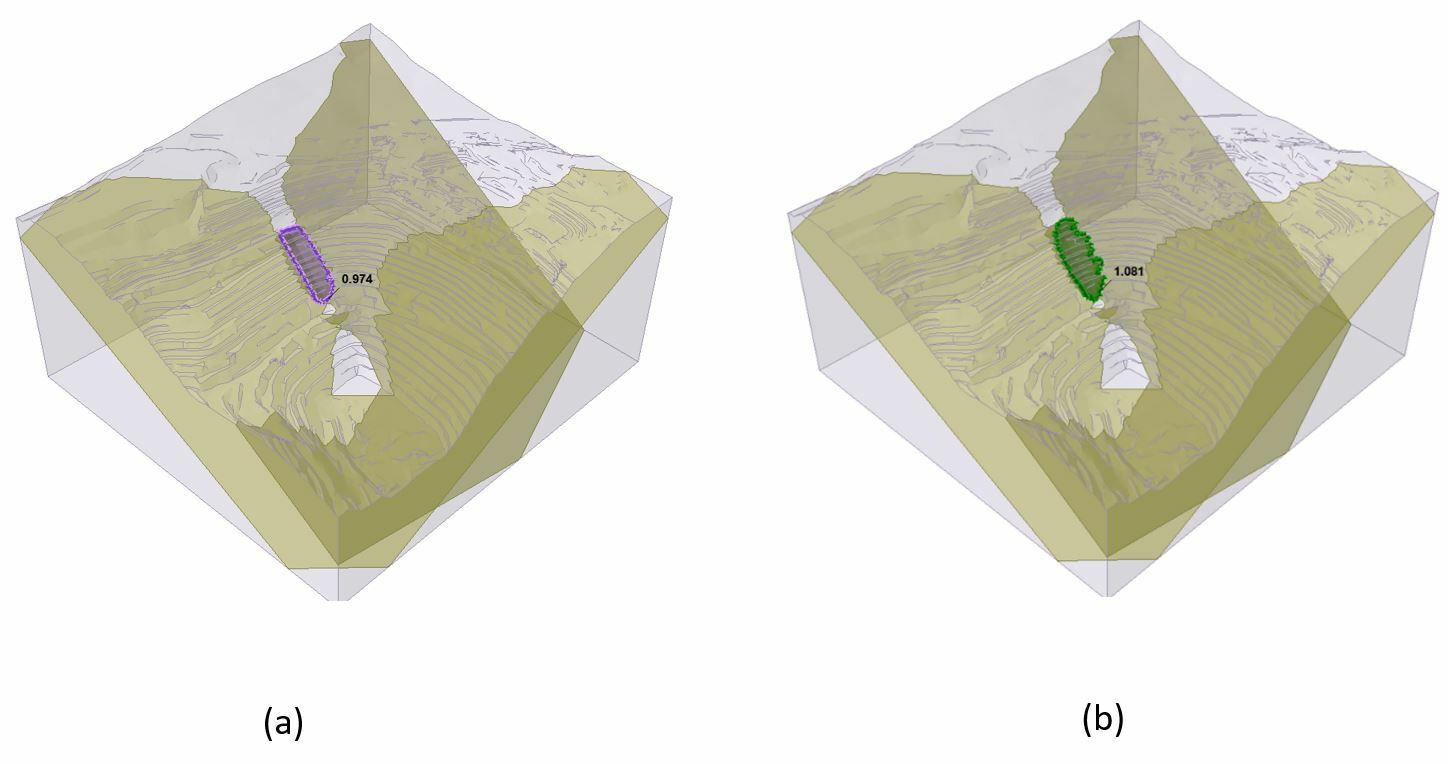Case Study

# Slope Stability Analysis of an Open Pit with Weak Layers, Using RS3 and Slide3

Published on: Nov. 23, 2022 Updated on: Nov. 09, 2023

The Limit Equilibrium Methods (LEMs) and Finite Element Methods (FEMs) are extensively used in slope stability analysis to identify critical failure surfaces. Slide3 uses the theories and principles of the LEM method, while RS3 is based on the FEM. Like the LEMs, RS3 can calculate factors of safety using the Shear Strength Reduction (SSR) technique. The program also computes stresses and deformations distribution in the domain.

The goal of the article is to compare the stability of an open pit in the presence of weak layers, using RS3 and Slide3. Even though each program uses a different approach, it has been shown in many scenarios (depending on the mechanism of failure), the critical failure path and factor of safety are very comparable. As such, these two programs can be used interchangeably.

## Model Geometry

An open pit, approximately 635m long, 600m wide, and 200m deep is used for this demonstration. The pit slopes have a bench width of 7m, bench height of 8 m, and bench face angle of 51o

The model consists of a single Mohr-Coulomb material and two planes of weaknesses dipping at 60 o with dip directions of 150 o and 220 o, respectively, as shown in Figure 1. The strength and deformation properties of the slope material and discontinuities are listed in Table 1 below.

Table 1: Material Properties

 Material Failure criterion C (kPa) Friction Angle E (kPa) v Normal Stiffness (kPa/m) Shear Stiffness (kPa/m) Fresh Rock Mohr-Coulomb 450 39 50000 0.4 - - Fault Mohr-Coulomb 20 20 - - 100000 10000

## Slide3 Analysis

The Janbu Simplified and Spencer methods were used to locate the critical slip surface in Slide3. These two methods were chosen because the Janbu Simplified method gives the lower bound factor of safety value, while the Spencer method satisfies complete equilibrium.

For both methods, the initial failure surface is set to be ellipsoid. To detect the critical slip surface, the cuckoo search algorithm and surface altering optimization is employed, so at the end, the failure surface wouldn’t necessarily be an ellipsoid shape.

Figure 2 shows the outcome of the Slide3 LEM analysis. The Janbu Simplified method gave a factor of safety of 0.97, while the Spencer method produced a factor of safety of 1.08.Figure 2: (a) critical failure surface for Janbu Method. (b) critical failure surfaces for Spencer Method

## RS3 Analysis

The Slide3 model was imported into RS3 and meshed with 10-noded tetrahedral elements. The bottom and all vertical sides of the model’s external boundary were pinned, i.e., restricted from moving. In RS3, the weak planes were modelled with joint elements with the material properties shown in Table1.

After running the model, the critical SRF (FS) was 1.11, which was comparable to that of the Spencer Method (1.08). The total displacement contours of the RS3 analysis, shown in Figure 3, identified a failure mechanism which compared well with Slide3.

To further verify the similarity of the results, the critical slip surfaces for both the Janbu Simplified and Spencer methods were superimposed on the total displacement contours of the RS3 model, shown in Figure 4 below.